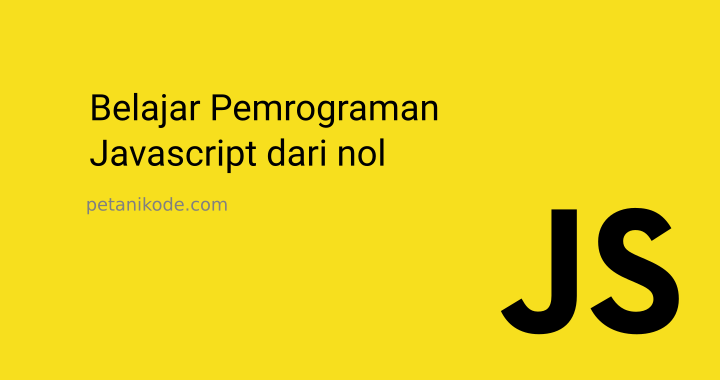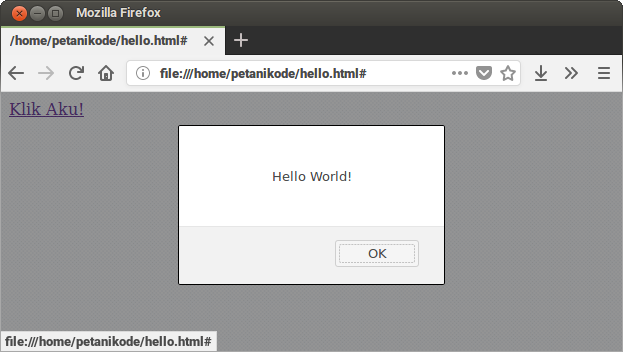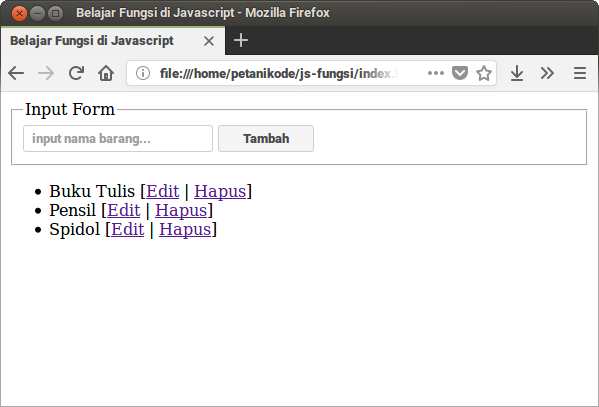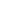| lesson material | material summary | questions and answers | definitions | types and examples | other information | materi pelajaran | ringkasan materi | pertanyaan dan jawaban | definisi | jenis-jenis dan contoh-contoh | informasi lainnya |

# Learning Javascript: Understanding Functions in Javascript and Program ExamplesIn programming, functions are often used to wrap programs into small parts.
The program logic in the function can be used again by calling it.
So there is no need to rewrite.
On this occasion, we will learn about functions in Javascript.
Starting from how to make, call, and make simple CRUD programs with functions.
Let's start…

## What is function?

Functions are sub-programs that can be reused both within the program itself and in other programs.
The function in Javascript is an object. Because it has properties and methods.
For beginners this concept is quite confusing. Moreover, those who are not familiar with the OOP concept .
We learn first about functions, then I will discuss about objects at different densities.

## 4 Ways to Make Functions in Javascript

There are 4 ways we can do a function in JavaScript:
1. Using the usual method;
2. Using expressions;
3. Using the arrow ( `=>`);
4. and use a constructor .
Let's try everything ...

### 1. Making Functions in the Ordinary Way

This method is most often used, especially for those who are just learning Javascript.
``````function namaFungsi(){
console.log("Hello World!");
}
``````

### 2. Making Functions with Expressions

How to create a function with an expression:
``````var namaFungsi = function(){
console.log("Hello World!");
}
``````
We use variables, then they are filled with functions. This function is actually an anonymous function or an anonymous function .

### 3. Make a Function with Arrows

This method is often used in today's Javascript code, because it is simpler. But it is difficult to understand for beginners. This function starts to appear in the ES6 standard.
Example:
``````var namaFungsi = () => {
console.log("Hello World!");
}

// atau seperti ini (jika isi fungsi hanya satu baris):
var namaFungsi = () => console.log("Hello World!");
``````
Actually it's almost the same as using expressions. The difference is, we use the arrow ( `=>`) instead `function`.
Making a function in this way is called an arrow function .

### 4. Making Functions with the Constructors

This method is actually not recommended by Mozilla Developer , because it looks less good. The problem is that body functions are made in string form which can affect the performance of the javascript engine .
Example:
``````var namaFungsi = new Function('console.log("Hello World!");');
``````
For those who are beginners, I recommend using the first method first. Later, when you get used to it, try using the 2nd and 3rd ways.

## How to Call / Execute Functions

After knowing how to make a function, then how do you call it?
We can call the function in the Javascript code by writing the name of the function like this:
``````namaFungsi();
``````
Example:
``````// membuat fungsi
function sayHello(){
console.log("Hello World!");
}

// memanggil fungsi
sayHello() // maka akan menghasilkan -> Hello World!
``````
In addition to the above method, we can also call functions through eventattributes in HTML.
Example:
``````<!DOCTYPE html>
<html>
<script>
// membuat fungsi
var sayHello = () => alert("Hello World!");
</script>
<body>
<!-- Memanggil fungsi saat link diklik -->
<a href="#" onclick="sayHello()">Klik Aku!</a>
</body>
</html>
``````
The result:## Functions with Parameters

Parameters are variables that store values ​​for processing in functions.
Example:
``````function kali(a, b){
hasilKali = a * b;
console.log("Hasil kali a*b = " + hasilKali);
}
``````
In the example above, `a`and `b`is a parameter.
Then how to call a function that has parameters like this:
``````kali(3, 2); // -> Hasil kali a*b = 6
``````
We provide `3`for parameters `a`and `2`for parameters `b`.

## Function that Returns Value

In order for the results of processing values ​​in functions to be used for the next process, the function must return a value.
Returns the value of the function using the keyword `return`then followed by the value or variable that will be returned. Example:
``````function bagi(a,b){
hasilBagi = a / b;
return hasilBagi;
}

// memanggil fungsi
var nilai1 = 20;
var nilai2 = 5;
var hasilPembagian = bagi(nilai1, nilai2);

console.log(hasilPembagian); //-> 4
``````

## Examples of Javascript Programs with Functions

After we understand the basics of making functions and types, now let's try to make a simple program.
This program contains CRUD (Crete, Read, Update, Delete) data items stored in an array.
``````js-fungsi/
├── fungsi.js
└── index.html
``````
Files `index.html`are files that display web pages. While the file `fungsi.js`is the program.
The following is the contents of the file `index.html`:
``````<!DOCTYPE html>
<html lang="en">
<meta charset="UTF-8">
<meta name="viewport" content="width=device-width, initial-scale=1.0">
<meta http-equiv="X-UA-Compatible" content="ie=edge">
<title>Belajar Fungsi di Javascript</title>
<body>

<fieldset>
<legend>Input Form</legend>
<input type="text" name="barang" placeholder="input nama barang..." />
</fieldset>

<div>
<ul id="list-barang">
</ul>
</div>

<script src="fungsi.js"></script>
</body>
</html>
``````
Next we will code in the file `fungsi.js`Please use the style of making functions that you like.
In this example, we will use the first method. Because it's easier.
The following is the contents of the file `fungsi.js`:
``````var dataBarang = [
"Buku Tulis",
"Pensil",
"Spidol"
];

function showBarang(){
var listBarang = document.getElementById("list-barang");
// clear list barang
listBarang.innerHTML = "";

// cetak semua barang
for(let i = 0; i < dataBarang.length; i++){
var btnEdit = "<a href='#' onclick='editBarang("+i+")'>Edit</a>";
var btnHapus = "<a href='#' onclick='deleteBarang("+i+")'>Hapus</a>";

listBarang.innerHTML += "<li>" + dataBarang[i] + " ["+btnEdit + " | "+ btnHapus +"]</li>";
}
}

var input = document.querySelector("input[name=barang]");
dataBarang.push(input.value);
showBarang();
}

function editBarang(id){
var newBarang = prompt("Nama baru", dataBarang[id]);
dataBarang[id] = newBarang;
showBarang();
}

function deleteBarang(id){
dataBarang.splice(id, 1);
showBarang();
}

showBarang();
``````
The result:##### 0 Komentar untuk "Learning Javascript: Understanding Functions in Javascript and Program Examples"

Silahkan berkomentar sesuai artikel

Template By Kunci Dunia